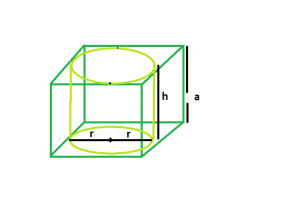# Largest right circular cylinder within a cube

Given a cube of side length a. The task is to find the volume of biggest right circular cylinder that can be inscribed within it.

Examples:

```Input :  a = 4
Output : 50.24

Input : a = 5
Output : 98.125
```

## Recommended: Please try your approach on {IDE} first, before moving on to the solution.Approach:
Let:

• The height of the cylinder is h.
• Radius of the cylinder be r.

From the diagram it is clear that:

• The height of the cylinder = side of cube
• Radius of the cylinder = side of the cube/2

So,

```h = a
r = a/2```

Below is the implementation of the above approach:

## C++

 `// C++ Program to find the biggest right ` `// circular cylinder that can be fit within a cube ` `#include ` `using` `namespace` `std; ` ` `  `// Function to find the biggest right circular cylinder ` `float` `findVolume(``float` `a) ` `{ ` `    ``// side cannot be negative ` `    ``if` `(a < 0) ` `        ``return` `-1; ` ` `  `    ``// radius of right circular cylinder ` `    ``float` `r = a / 2; ` ` `  `    ``// height of right circular cylinder ` `    ``float` `h = a; ` ` `  `    ``// volume of right circular cylinder ` `    ``float` `V = 3.14 * ``pow``(r, 2) * h; ` ` `  `    ``return` `V; ` `} ` ` `  `// Driver code ` `int` `main() ` `{ ` `    ``float` `a = 5; ` ` `  `    ``cout << findVolume(a) << endl; ` ` `  `    ``return` `0; ` `} `

## Java

 `// Java Program to find the biggest right ` `// circular cylinder that can be fit within a cube ` ` `  `import` `java.io.*; ` ` `  `class` `GFG { ` `   `  ` `  `// Function to find the biggest right circular cylinder ` ` ``static` `float` `findVolume(``float` `a) ` `{ ` `    ``// side cannot be negative ` `    ``if` `(a < ``0``) ` `        ``return` `-``1``; ` ` `  `    ``// radius of right circular cylinder ` `    ``float` `r = a / ``2``; ` ` `  `    ``// height of right circular cylinder ` `    ``float` `h = a; ` ` `  `    ``// volume of right circular cylinder ` `    ``float` `V = (``float``)(``3.14` `* Math.pow(r, ``2``) * h); ` ` `  `    ``return` `V; ` `} ` ` `  `// Driver code ` ` `  ` `  `    ``public` `static` `void` `main (String[] args) { ` `            ``float` `a = ``5``; ` ` `  `    ``System.out.print(findVolume(a)); ` `    ``} ` `} ` `// This code is contributed by anuj_67.. `

## Python3

 `# Python3 Program to find the biggest  ` `# right circular cylinder that can be  ` `# fit within a cube  ` ` `  `# Function to find the biggest right ` `# circular cylinder  ` `def` `findVolume(a) : ` ` `  `    ``# side cannot be negative  ` `    ``if` `(a < ``0``) :  ` `        ``return` `-``1` ` `  `    ``# radius of right circular cylinder  ` `    ``r ``=` `a ``/` `2` ` `  `    ``# height of right circular cylinder  ` `    ``h ``=` `a ` ` `  `    ``# volume of right circular cylinder  ` `    ``V ``=` `3.14` `*` `pow``(r, ``2``) ``*` `h  ` ` `  `    ``return` `V  ` ` `  `# Driver code  ` `if` `__name__ ``=``=` `"__main__"` `:  ` ` `  `    ``a ``=` `5` ` `  `    ``print``(findVolume(a)) ` ` `  `# This code is contributed by Ryuga `

## C#

 `// C# Program to find the biggest right ` `// circular cylinder that can be fit within a cube ` ` `  `using` `System; ` `class` `GFG { ` ` `  ` `  `// Function to find the biggest right circular cylinder ` `static` `float` `findVolume(``float` `a) ` `{ ` `    ``// side cannot be negative ` `    ``if` `(a < 0) ` `        ``return` `-1; ` ` `  `    ``// radius of right circular cylinder ` `    ``float` `r = a / 2; ` ` `  `    ``// height of right circular cylinder ` `    ``float` `h = a; ` ` `  `    ``// volume of right circular cylinder ` `    ``float` `V = (``float``)(3.14 * Math.Pow(r, 2) * h); ` ` `  `    ``return` `V; ` `} ` ` `  `// Driver code ` ` `  ` `  `    ``public` `static` `void` `Main () { ` `            ``float` `a = 5; ` ` `  `   ``Console.WriteLine(findVolume(a)); ` `    ``} ` `} ` `// This code is contributed by anuj_67.. `

## PHP

 `

Output:

```98.125
```

Don’t stop now and take your learning to the next level. Learn all the important concepts of Data Structures and Algorithms with the help of the most trusted course: DSA Self Paced. Become industry ready at a student-friendly price.

My Personal Notes arrow_drop_upCheck out this Author's contributed articles.

If you like GeeksforGeeks and would like to contribute, you can also write an article using contribute.geeksforgeeks.org or mail your article to contribute@geeksforgeeks.org. See your article appearing on the GeeksforGeeks main page and help other Geeks.

Please Improve this article if you find anything incorrect by clicking on the "Improve Article" button below.

Improved By : vt_m, AnkitRai01, Akanksha_Rai